HOMER Grid 1.8

 Navigation: HOMER's Calculations How HOMER Calculates the PV Cell TemperatureThe photovoltaic (PV) cell temperature is the temperature of the surface of the PV array. During the night, it is the same as the ambient temperature, but in full sun, the cell temperature can exceed the ambient temperature by 30°C or more.

If, in the PV array inputs, you choose to consider the effect of temperature on the PV array, HOMER calculates the cell temperature in each time step and use it in calculating the power output of the PV array. The following describes how HOMER calculates the cell temperature from the ambient temperature and the radiation striking the array.

We start by defining an energy balance for the PV array, using the following equation from Duffie and Beckman (1991):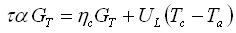where: τ = the solar transmittance of any cover over the PV array [%] α = the solar absorptance of the PV array [%] GT = the solar radiation striking the PV array [kW/m2] ηc = the electrical conversion efficiency of the PV array [%] UL = the coefficient of heat transfer to the surroundings [kW/m2°C] Tc = the PV cell temperature [°C] Ta = the ambient temperature [°C]

The equation above states that a balance exists between, on one hand, the solar energy absorbed by the PV array, and on the other hand, the electrical output plus the heat transfer to the surroundings. We can solve that equation for cell temperature to yield:It is difficult to measure the value of (??/UL) directly, so instead manufacturers report the nominal operating cell temperature (NOCT), which is defined as the cell temperature that results at an incident radiation of 0.8 kW/m2, an ambient temperature of 20°C, and no load operation (meaning ηc = 0). We can substitute these values into the equation above and solve it for ??/UL to yield the following equation: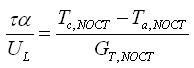where: Tc,NOCT = the nominal operating cell temperature [°C] Ta,NOCT = the ambient temperature at which the NOCT is defined [20°C] GT,NOCT = the solar radiation at which the NOCT is defined [0.8 kW/m2]

If we assume that τα/UL is constant, we can substitute this equation into the cell temperature equation to yield: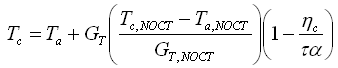HOMER assumes a value of 0.9 for τα in the equation above, as Duffie and Beckman (1991) suggest. Because the term ηc/τα is small compared to unity, this assumption does not introduce significant error.

HOMER assumes that the PV array always operates at its maximum power point, as it does when controlled by a maximum power point tracker. That means HOMER assumes the cell efficiency is always equal to the maximum power point efficiency: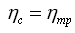where: ηmp = the efficiency of the PV array at its maximum power point [%]

So in the equation for cell temperature, we can replace ηc with ηmp to yield: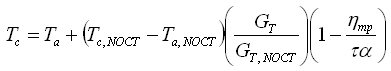But ηmp depends on the cell temperature Tc. HOMER assumes that the efficiency varies linearly with temperature according to the following equation: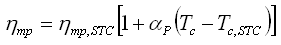where: ηmp,STC αP = the temperature coefficient of power [%/°C] Tc,STC = the cell temperature under standard test conditions [25°C]

The temperature coefficient of power is normally negative, meaning that the efficiency of the PV array decreases with increasing cell temperature.

We can substitute this efficiency equation into the preceding cell temperature equation and solve for cell temperature to yield: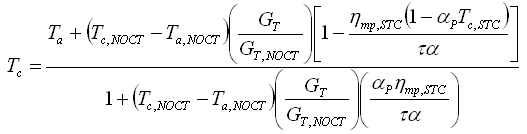The temperatures in the equation above must be in Kelvin. HOMER uses this equation to calculate the cell temperature in each time step.